Conditional Probability - Statement

Chapter 13 Class 12 Probability
Concept wise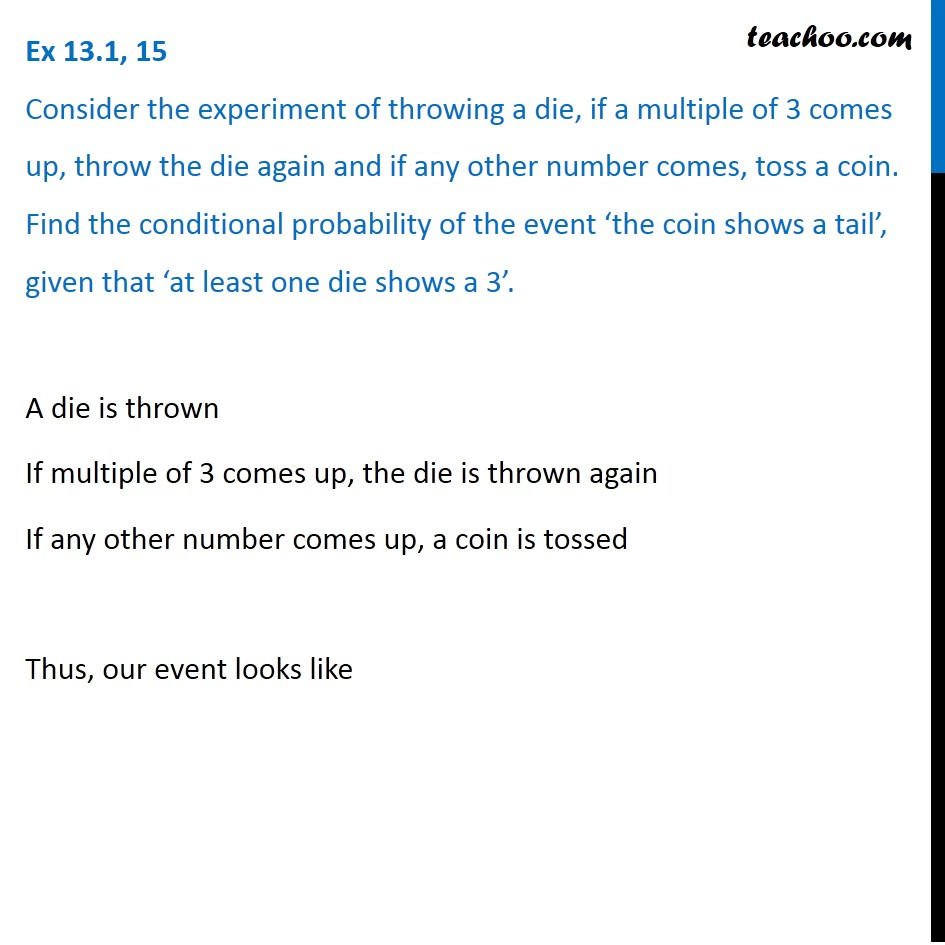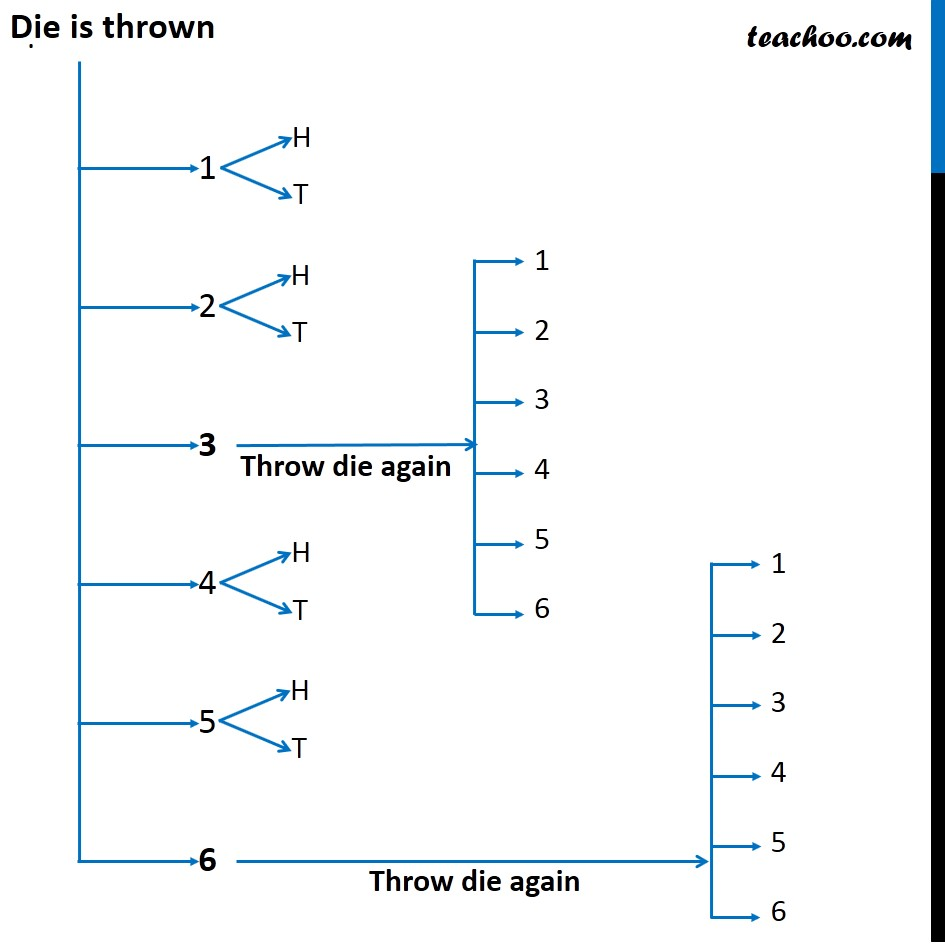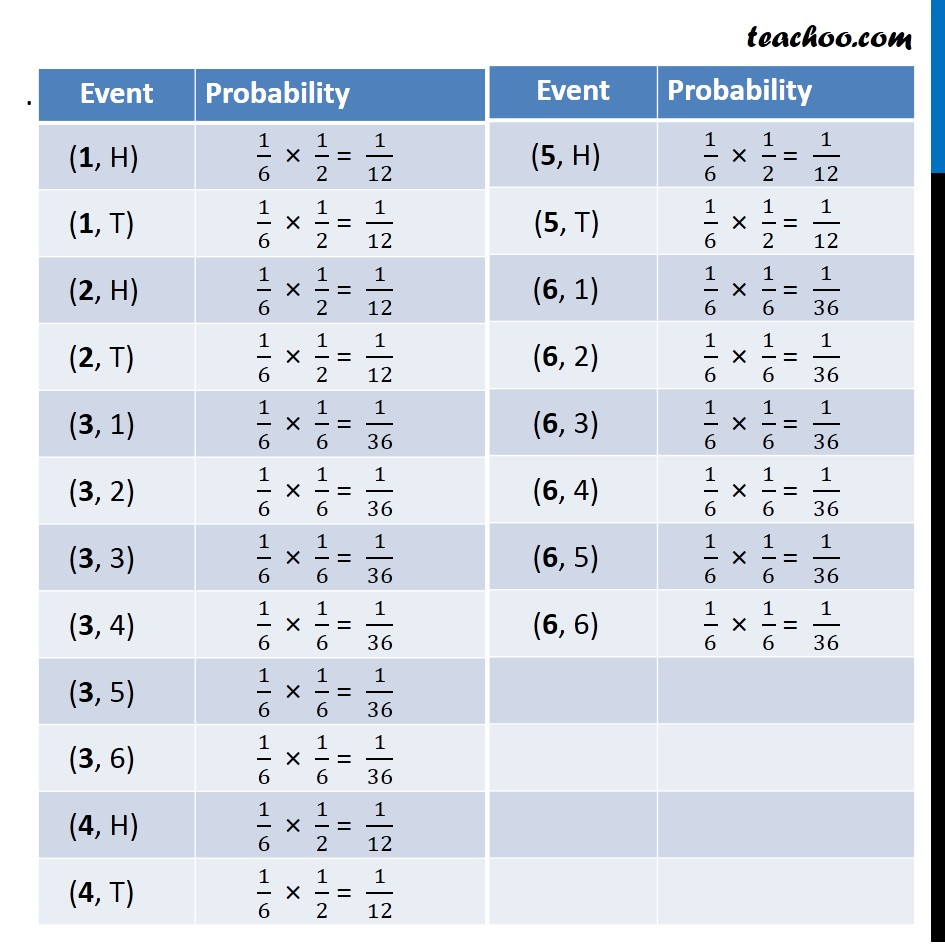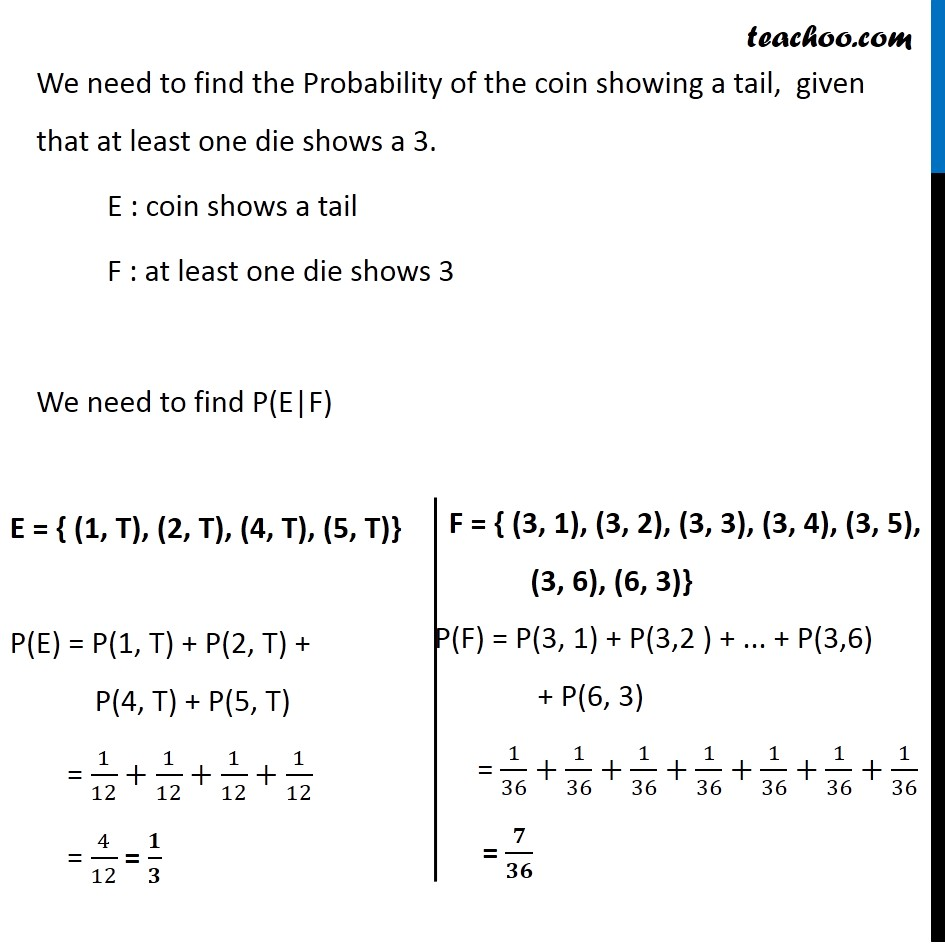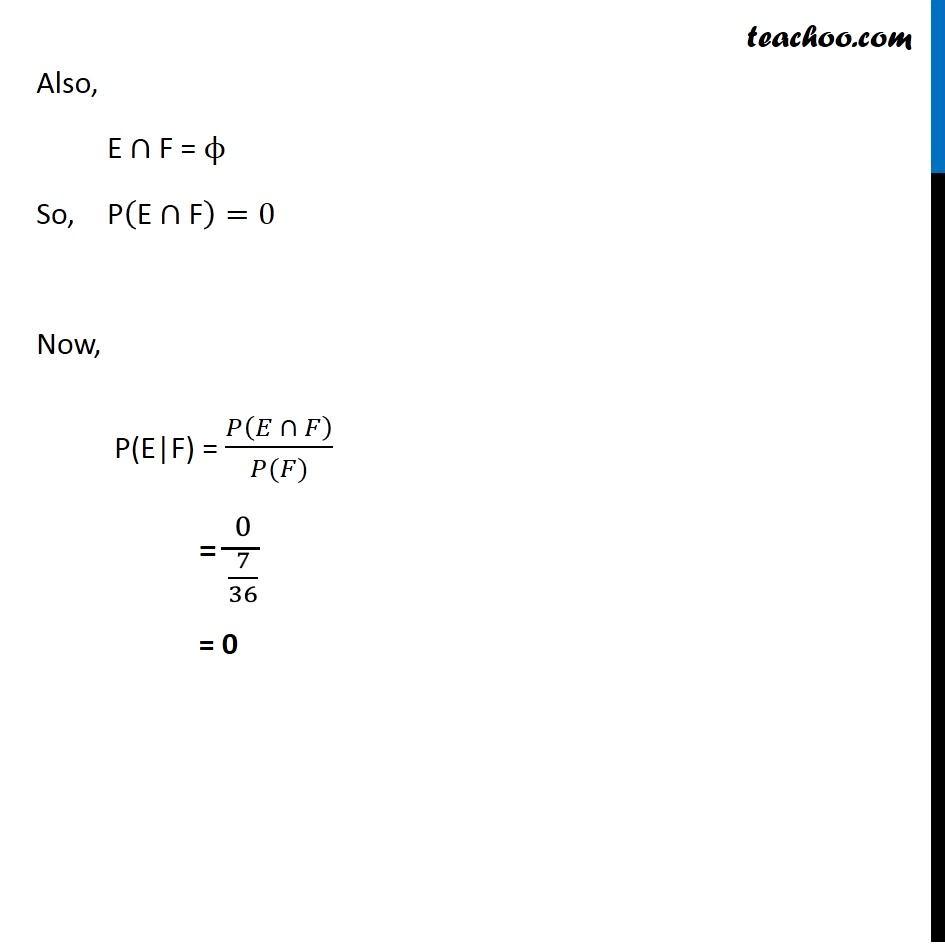Learn in your speed, with individual attention - Teachoo Maths 1-on-1 Class

### Transcript

Ex 13.1, 15 Consider the experiment of throwing a die, if a multiple of 3 comes up, throw the die again and if any other number comes, toss a coin. Find the conditional probability of the event ‘the coin shows a tail’, given that ‘at least one die shows a 3’.A die is thrown If multiple of 3 comes up, the die is thrown again If any other number comes up, a coin is tossed Thus, our event looks like We need to find the Probability of the coin showing a tail, given that at least one die shows a 3. E : coin shows a tail F : at least one die shows 3 We need to find P(E|F) E = { (1, T), (2, T), (4, T), (5, T)} P(E) = P(1, T) + P(2, T) + P(4, T) + P(5, T) = 1/12+1/12+1/12+1/12 = 4/12 = 𝟏/𝟑 F = { (3, 1), (3, 2), (3, 3), (3, 4), (3, 5), (3, 6), (6, 3)} P(F) = P(3, 1) + P(3,2 ) + ... + P(3,6) + P(6, 3) = 1/36+1/36+1/36+1/36+1/36+1/36+1/36 = 𝟕/𝟑𝟔 Also, "E"∩"F" = ϕ So, P("E" ∩"F" )=0 Now, P(E|F) = 𝑃(𝐸 ∩ 𝐹)/(𝑃(𝐹)) = 0/(7/36) = 0